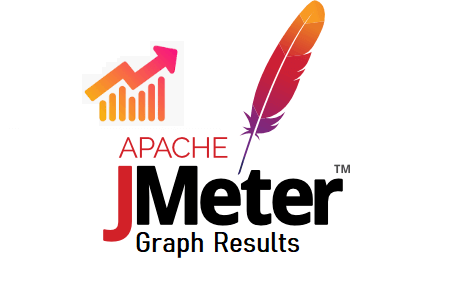JMeter – Graph ResultsIn JMeter, Graph Results Listener plots a graph between response time in milliseconds and elapsed time. The graph lines show the response time of all the requests (Data), the average response time (Average), the median of response time (Median), deviation in the response time (Deviation) and no. of samples per minute (throughput). The summarized figures can be seen at the bottom of the graph.

1. Select the appropriate element where you want to add the listener
1. Test Plan